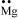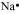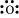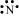The Lewis dot symbols for atoms of the elements Mg, Na, O, and N, respectively, would be:

 1234Subtopic:  Octate, Isoelectronic Species, Lewis Structure & Formal Charge |
84%
From NCERT
To view explanation, please take trial in the course.
NEET 2023 - Target Batch - Aryan Raj Singh
Hints
To view explanation, please take trial in the course.
NEET 2023 - Target Batch - Aryan Raj Singh

Lewis symbols for the atoms and ions: S and S2–; Al and Al3+; H and H respectively, would be:

 1${\left[\mathbf{:}\underset{\mathbf{.}\mathbf{.}}{\overset{\mathbf{.}\mathbf{.}}{\mathrm{S}}}\mathbf{:}\right]}^{2-}$ ${\left[\mathrm{Al}\right]}^{3+}$2${\left[\mathbf{:}\underset{\mathbf{.}\mathbf{.}}{\overset{\mathbf{.}\mathbf{.}}{\mathrm{S}}}\mathbf{:}\right]}^{2-}$${\left[\mathrm{Al}\right]}^{3+}$3 ${\left[\mathbf{:}\underset{\mathbf{.}\mathbf{.}}{\overset{\mathbf{.}\mathbf{.}}{\mathrm{S}}}\mathbf{:}\right]}^{2-}$${\left[\mathrm{Al}\right]}^{3+}$4${\left[\mathbf{:}\underset{\mathbf{.}\mathbf{.}}{\overset{\mathbf{.}\mathbf{.}}{\mathrm{S}}}\mathbf{:}\right]}^{2-}$${\left[\mathrm{Al}\right]}^{3+}$Subtopic:  Octate, Isoelectronic Species, Lewis Structure & Formal Charge |
79%
From NCERT
To view explanation, please take trial in the course.
NEET 2023 - Target Batch - Aryan Raj Singh
Hints
To view explanation, please take trial in the course.
NEET 2023 - Target Batch - Aryan Raj Singh

The lone pairs of electrons can be defined as -

1. Electron pairs that participate in bonding.

2. Electron pairs  that do not participate in bonding.

3. Electron pairs that are present in inner most shell.

4.Electron pairs that are present in valence shell of ions.Subtopic:  Octate, Isoelectronic Species, Lewis Structure & Formal Charge |
84%
From NCERT
To view explanation, please take trial in the course.
NEET 2023 - Target Batch - Aryan Raj Singh
Hints
To view explanation, please take trial in the course.
NEET 2023 - Target Batch - Aryan Raj Singh

The significance of the octet rule is:

1. To determine the stability of atoms.

2. To find the hybridization of elements.

3. To calculate the number of lone pairs.

4. None of the above.Subtopic:  Octate, Isoelectronic Species, Lewis Structure & Formal Charge |
72%
From NCERT
To view explanation, please take trial in the course.
NEET 2023 - Target Batch - Aryan Raj Singh
Hints
To view explanation, please take trial in the course.
NEET 2023 - Target Batch - Aryan Raj Singh

The correct Lewis structure of acetic acid is -

 1234 None of the above.Subtopic:  Octate, Isoelectronic Species, Lewis Structure & Formal Charge |
83%
From NCERT
To view explanation, please take trial in the course.
NEET 2023 - Target Batch - Aryan Raj Singh
Hints